# Lattice and CrystalAn ideal crystal is a repetition of identical structural units in three dimensional space. The periodicity is described by a mathematical lattice (which are mathematical points at specific coordinates in space), the identical structural units (or base of the crystal) are the atoms in some specific arrangement which are unambiguously placed at every lattice point. Note that a lattice is not a crystal, even so the two words are often used synonymously in colloquial language, especially in the case of elemental crystals where the base consists of one atom only.All possible lattices can be described by a set of three linearly independent vectors a1, a2, and a3, the unit vectors of the lattice. Each lattice point than can be reached by a translation vector T of the lattice given by
T  =  (u · a1, v · a1, w · a3)With u, v, w = integers.It is convenient, to classify lattices according to some basic symmetry groups. This yields the 14 Bravais lattices, which are commonly used to describe lattice types. Their basic features are shown below (For sake of clarity, the lattice points are shown as little spheres and occasionally only "visible" lattice points are shown. These are not atoms, however!)
Name of crystal system Angles Cubic Length of Base vectors between axes a1= a2 = a3 Bravais Lattices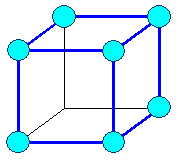cubic primitive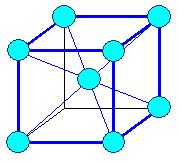cubic body centered (bcc)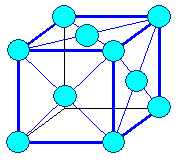cubic face centered (fcc)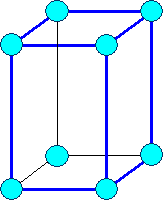Tetragonal primitive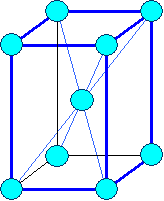Tetragonal body centered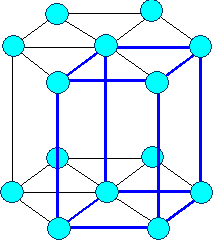Hexagonal (elementary cell continued to show hex. symmetry)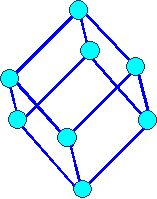Rhombohedral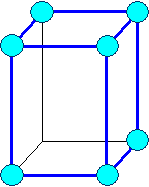Orthorhombic primitive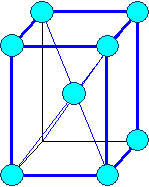Orthorhombic body centered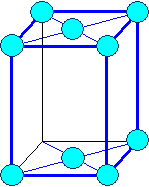Orthorhombic base face centered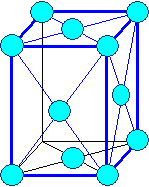Orthorhombic face centered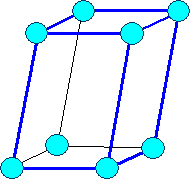Monocline primitive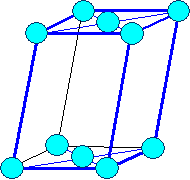Monocline base face centered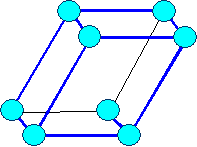TriclineA crystal now is obtained by taking a Bravais lattice and adding a base!The base can just be one atom (as in the case of many elemental crystals, most noteworthy the metals), two identical atoms (e.g. Si, Ge, C(diamond)), two different atoms (NaCl, GaAs, ...) three atoms, ... up to huge complex molecules as in the case of protein crystals.An arbitrary example is shown below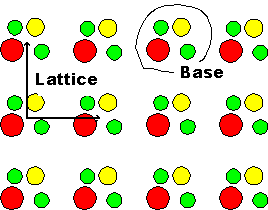For certain applications, a Bravais lattice may not be the best choice. Whereas, for example, it shows best the cubic symmetry of the cubic lattices, its elementary cell is not a primitive unit cell of the lattice, i.e. there are unit cells with a smaller volume (but without the cubic symmetry). For other cases (especially if working in reciprocal lattices) the choice of a Wigner-Seitz cell may be appropriate, which is obtained by intersecting all lines from one lattice point to neighboring points at half the distance with planes at right angles to the linesThis is shown schematically below: The blue lines connect lattice points, the red lines denote the intersection at right angles. The resulting Wigner-Seitz cell and its use in constructing the lattice are shown in yellow.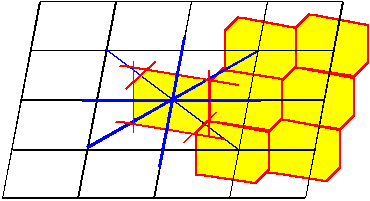In practical work, one oftens refers to crystal types instead of lattices by using the name of prominent crystals, crystallographers or minerals etc.; e.g. "diamond type, Perovskites, "Zinkblende" structure and so on. A few examples are given in the link.1.3.3 The larger View and Complications2.1.1 Simple Vacancies and InterstitialsIonic Crystals5.1.1 Dislocations5.4.1 Partial Dislocations and Stacking Faults7.3.2 Working with the O-LatticeExercise 3.1-1: Calculate the Geometry FactorStacking Faults in the DSC LatticeSolution to Exercise 3.1-1

© H. Föll (Defects - Script)